# Relational Comparisons

 In Chapter 2, I mentioned the fact that the equality comparison operator "=" applies to every type. In particular, therefore, it applies to relation types; that is, given two relations r and s of the same relation type T, we must at least be able to test whether those two relations are equal. Other comparisons might be useful, too; for example, we might want to test whether r is a subset of s (meaning every tuple in r is also in s), or whether r is a proper subset of s (meaning every tuple in r is also in s, but s contains at least one tuple that isn't in r). Now, I must immediately explain that these operators aren't relational operators as such that is, they're not part of the relational algebra because their result is a truth value, not a relation. But it's convenient to discuss them in this chapter, and I will. Here's a simple example: ` S { CITY } = P { CITY } ` The left comparand is the projection of suppliers on CITY, the right comparand is the projection of parts on CITY, and the comparison returns TRUE if these two projections are equal, FALSE otherwise. In other words, the comparison (which is, of course, a boolean expression) means: "Is the set of supplier cities equal to the set of part cities?" Here's another example: ` S { SNO }SP { SNO } ` Explanation: The symbol "" here means "is a proper superset of." The meaning of this comparison (considerably paraphrased) is: "Are there any suppliers who supply no parts at all?" Other useful relational comparison operators include "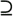" ("is a superset of), "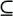" ("is a subset of), and "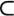" ("is a proper subset of). The obvious place where relational comparisons are useful is in connection with restrictions.[*] Let's look at some examples. Consider first the query "Get suppliers who supply all parts." Here's a possible formulation:[*] But see Exercise 5-26 at the end of the chapter. ` WITH ( SP RENAME ( SNO AS X ) ) AS R : S WHERE ( R WHERE X = SNO ) { PNO } = P { PNO } ` Explanation: The expression WITH . . . AS R is effectively equivalent to an assignment that assigns the value of the expression SP RENAME (SNO AS X) to some temporary, system-generated relvar R; after that assignment, the value of R is a relation that's identical to the current value of relvar SP, except that attribute SNO has been renamed as X.[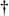] (The purpose of introducing the names R and X is simply to avoid a naming conflict that would subsequently arise otherwise.) Then, for a given supplier S [] SQL supports WITH too, though it writes the operands the other way around: WITH expression. ` ( R WHERE X = SNO ) { PNO } ` evaluates to a relation with one attribute (PNO), giving part numbers for all parts supplied by supplier S x. Note in particular that if supplier S x supplies no parts, that relation will contain no tuples. Finally, that degree-one relation is tested for equality with the relation that's the projection of P on PNO. Clearly, that test will give TRUE if and only if the set of part numbers for parts supplied by S x is equal to the set of part numbers in relvar P. The overall result thus contains precisely those tuples from relvar S that represent suppliers who supply all of the parts mentioned in relvar P. Of course, we can write the entire query as a single expression if we like: ` S WHERE ( ( SP RENAME ( SNO AS X ) ) WHERE X = SNO ) { PNO } = P { PNO } ` But using WITH to introduce names for the results of subexpressions often helps to simplify the job of formulating complicated queries. Here's a definition: if rx is a relational expression that mentions some relvar R, then WITH ry AS R: rx, where ry is another relational expression, is also a relational expression. Of course, rx might be a WITH expression, too, like this: ` WITH ry AS R1 : WITH rz AS R2 : rx ` This latter expression can be abbreviated to just: ` WITH ry AS R1, rz AS R2 : rx ` We'll see plenty of examples of this abbreviated form later. By the way, the foregoing example ("Get suppliers who supply all parts") is very similar to one that's often used to illustrate the use of the relational divide operator. To be specific, the expression: ` SP { SNO, PNO } DIVIDEBY P { PNO } ` (which I gave as an example of divide in the earlier section "The Original Operators") is often characterized as a relational formulation of the query "Get supplier numbers for suppliers who supply all parts." But it isn't! Rather, it is a relational formulation of the query "Get supplier numbers for suppliers who supply at least one part and in fact supply all parts." (If you're wondering what the logical difference is here, see Exercise 5-25 at the end of this chapter.) In my opinion, the formulation involving a relational comparison is not only easier to understand than the divide formulation it also has the advantage of being correct. Here's another example. The query is: "Get pairs of supplier numbers, S x and S y say, such that S x and S y supply exactly the same set of parts each." This query is very difficult without relational comparisons! Here it is: ` WITH ( SP RENAME ( SNO AS SX ) ) { SX, PNO } AS R1 , ( SP RENAME ( SNO AS SY ) ) { SY, PNO } AS R2 , R1 { SX } AS R3 , R2 { SY } AS R4 , ( R1 JOIN R4 ) AS R5 , ( R2 JOIN R3 ) AS R6 , ( R1 JOIN R2 ) AS R7 , ( R3 JOIN R4 ) AS R8 , SP { PNO } AS R9 , ( R8 JOIN R9 ) AS R10 , ( R10 MINUS R7 ) AS R11 , ( R6 JOIN R11 ) AS R12 , ( R5 JOIN R11 ) AS R13 , R12 { SX, SY } AS R14 , R13 { SX, SY } AS R15 , ( R14 UNION R15 ) AS R16 , R7 { SX, SY } AS R17 : R17 MINUS R16 ` But with relational comparisons it's fairly straightforward: ` WITH ( S RENAME ( SNO AS SX ) ) { SX } AS RX , ( S RENAME ( SNO AS SY ) ) { SY } AS RY : ( RX JOIN RY ) WHERE ( SP WHERE SNO = SX ) { PNO } = ( SP WHERE SNO = SY ) { PNO } ` As an aside, I remark that appending "AND SX < SY" to the WHERE clause here would produce a slightly tidier result; to be specific, it would (a) eliminate pairs of the form (S x,Sx) and (b) ensure that the pairs (Sx,Sy) and (Sy,Sx) don't both appear. One particular comparison that's often needed in practice is an "=" comparison between a given relation r and an empty relation of the same type in other words, a test to see whether r is empty. So let me introduce the following shorthand: ` IS_EMPTY ( rx ) ` This expression is defined to return TRUE if the relation r denoted by the relational expression rx is empty and FALSE otherwise. I'll be relying heavily on this construct in the next chapter. Another common requirement is to be able to test whether a given tuple t appears in a given relation r: ` t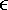This expression is defined to be shorthand for the relational comparison: RELATION { t }The left operand here is a relation selector invocation, and it returns a relation containing just the specified tuple t. The comparison thus returns TRUE if t appears in the relation r denoted by the relational expression rx and FALSE otherwise ("" is the tt[appears] in r"). In fact, as you've probably realized, the "" operator is essentially SQLs IN operator except that the left operand of SQL's IN is usually a scalar, not a row, which means there's some kind of coercion going on. Be that as it may, SQL certainly doesn't support any relational comparisons apart from this one rather special one. (Well, it does support NOT IN; so does Tutorial D, in the form of "".) `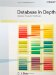Database in Depth: Relational Theory for Practitioners
ISBN: 0596100124
EAN: 2147483647
Year: 2006
Pages: 127
Authors: C.J. Date

Similar book on Amazon